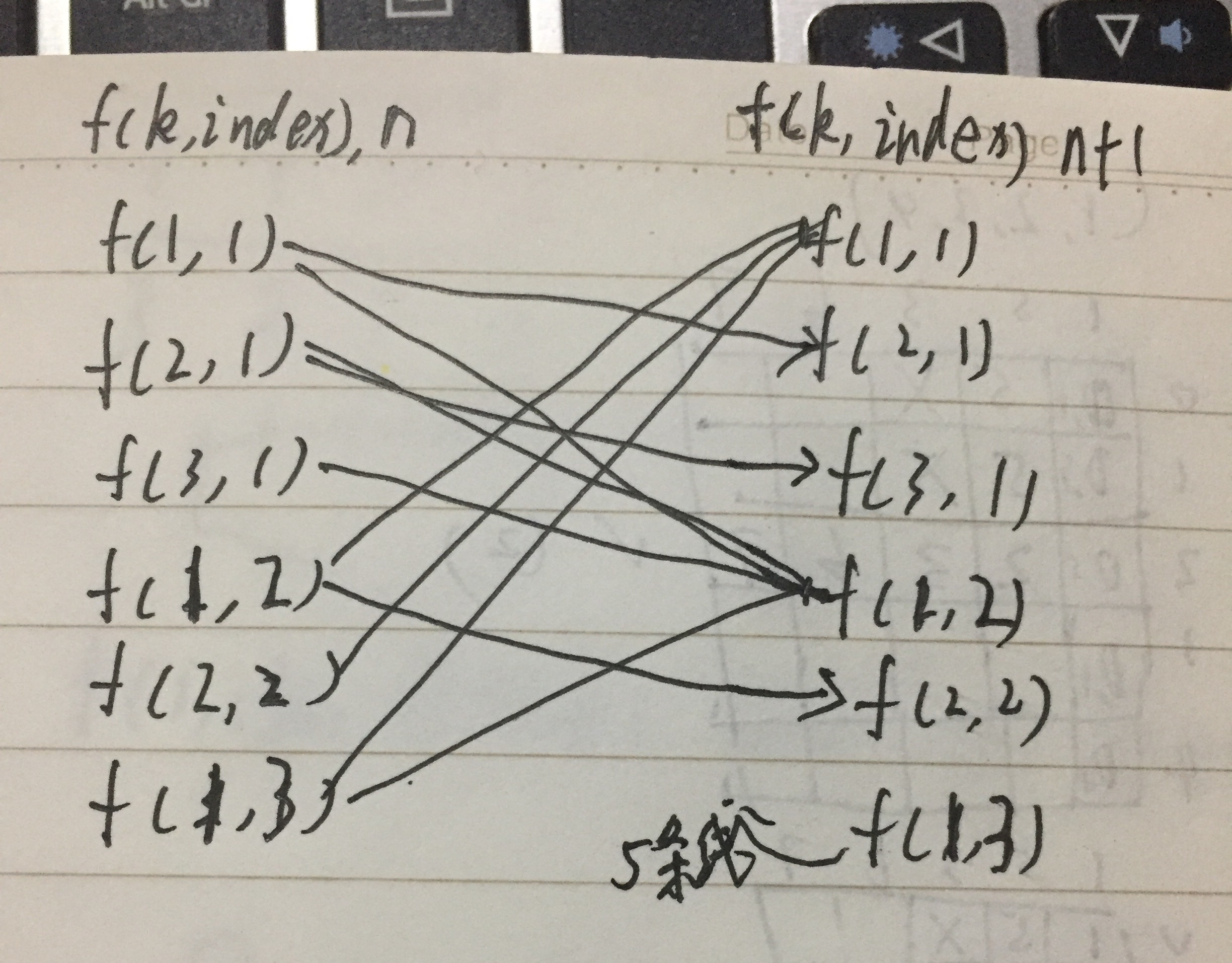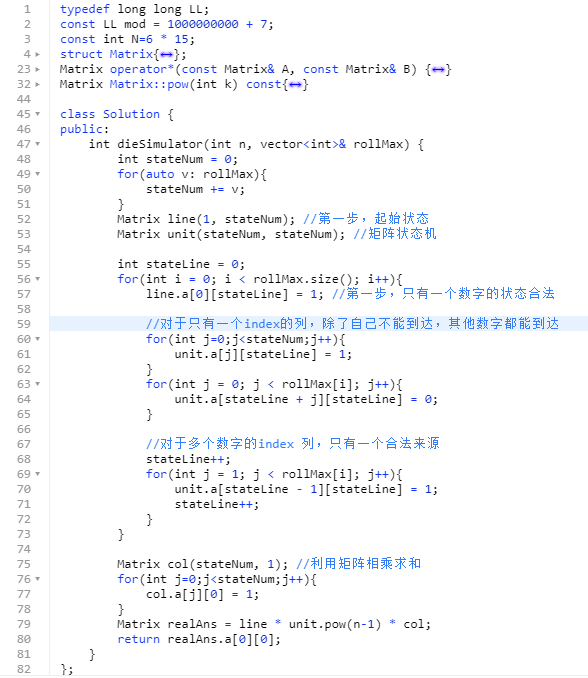# 有限制的随机数（矩阵状态机）

## 三、矩阵状态机`n+1`轮的`f(1,1)`，只能来自第`n`轮的不以`1`为后缀的所有状态答案之和。
`n+1`轮的`f(2,1)`，只能来自第`n`轮的`f(1,1)`的状态答案。

``````0: f(1, 1)
1: f(2, 1)
2: f(1, 2)
3: f(2, 2)
``````

``````  | 0 1 2 3
- - - - - -
0 | 0 1 1 0
1 | 0 0 1 0
2 | 1 0 0 1
3 | 1 0 0 0
``````

``````  | 0 1 2 3
- - - - - -
0 | 1 0 1 0
``````## 四、矩阵幂优化

``````2 ^ 15
= 2 ^ (1 + 2 + 4 + 8)
= 2^1 * 2^2 * 2^4 * 2^8

2^8 = 2^4 * 2^4
2^4 = 2^2 * 2^2
2^2 = 2^1 * 2^1
``````## 五、最后

1）当前状态只与上一个状态有关系
2）状态的维度很小
3）状态转移的次数很大时，递推会超时

-EOF-

## 关注公众号,接收最新消息

1.